# Electromagnetic Waves MCQs

The two components of the electromagnetic wave are the electric field and magnetic field. The electric field is formed due to the flow of voltage and the magnetic field from the flow of current.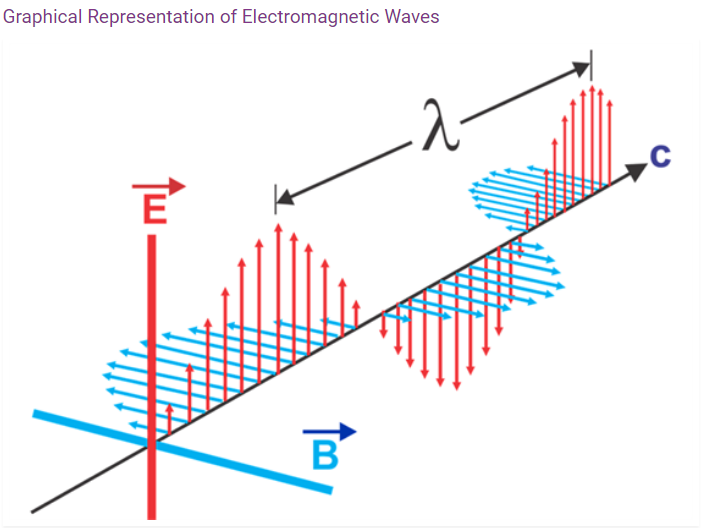Characteristics of Electromagnetic Wave

1. Electromagnetic waves are transverse in nature. The electric and magnetic field are perpendicular to each other.
2. Accelerated charges produce electromagnetic waves.
3. These waves travel at a constant velocity of 3 x 108 m/s in a vacuum.
4. Electromagnetic waves do not require a medium to travel.
5. The frequency of the wave remains unchanged but wavelength changes when it travels from one medium to another.
6. It follows the law of superposition.
7. The oscillating electric and magnetic fields are in phase and their magnitudes have a constant ratio.
8. The electric energy is equal to the magnetic energy in electromagnetic waves.
9. The Poynting vector represents the energy transferred by electromagnetic waves per unit area.

Q1: Electromagnetic waves are produced by

1. A static charge
2. An accelerated charge
3. A moving charge
4. Charged particles

Q2: The direction in which electromagnetic waves propagate is the same as that of

1.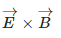2.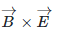3.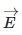4.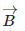Answer: (a)Q3: In electromagnetic waves the phase difference between electric field vector and magnetic field vector is

1. zero
2. π/2
3. π
4. π/3

Q4: In an electromagnetic wave in free space, the root mean square value of the electric field is 6 V/m. The peak value of the magnetic field is

1. 2.83 x 10-8 T
2. 1.51 x 10-8 T
3. 0.80 x 10-8 T
4. 4 x 10-8 T

Answer: (a) 2.83 x 10-8 T

Q5: Which of the following can be used to produce a propagating electromagnetic wave?

1. Charge moving at a constant speed
2. Chargeless particle
3. Stationary charge
4. An accelerating charge

Q6: To which part of the spectrum does EM waves belong if the energy of the wave is of the order of 15 KeV.

1. X rays
2. Infrared rays
3. Ultraviolet rays
4. Gamma rays

Q7: The ratio of the amplitude of the magnetic field to the amplitude of the electric field for electromagnetic wave propagation in a vacuum is equal to

1. Unity
2. Speed of light in vacuum
3. Reciprocal of the speed of light in vacuum
4. The ratio of magnetic permeability to electrical susceptibility in a vacuum.

Answer: (c) Reciprocal of the speed of light in vacuum

Q8: Which properties amount the following is false about electromagnetic waves?

1. The energy in an electromagnetic wave is divided equally between electric and magnetic vectors.
2. Both electric and magnetic field vectors are parallel to each other and perpendicular to the direction of propagation of the wave.
3. These waves do not require any material medium for propagation
4. Both electric and magnetic field vectors attain the maxima and minima at the same place and the same time

Answer: (b) Both electric and magnetic field vectors are parallel to each other and perpendicular to the direction of propagation of the wave.

Q9: The velocity of electromagnetic radiation in a medium of permittivity ε0 and permeability μ0 is given by

1. $$\sqrt{\varepsilon_{0}/\mu_{0}}$$
2. $$\sqrt{\varepsilon_{0}\mu_{0}}$$
3. 1/$$\sqrt{\varepsilon_{0}\mu_{0}}$$
4. $$\sqrt{\mu_{0}/\varepsilon_{0}}$$

Answer: (c) 1/$$\sqrt{\varepsilon_{0}\mu_{0}}$$

Q10: Which of the following rays are not electromagnetic waves?

1. Gamma rays
2. Beta rays
3. Heat rays
4. X rays